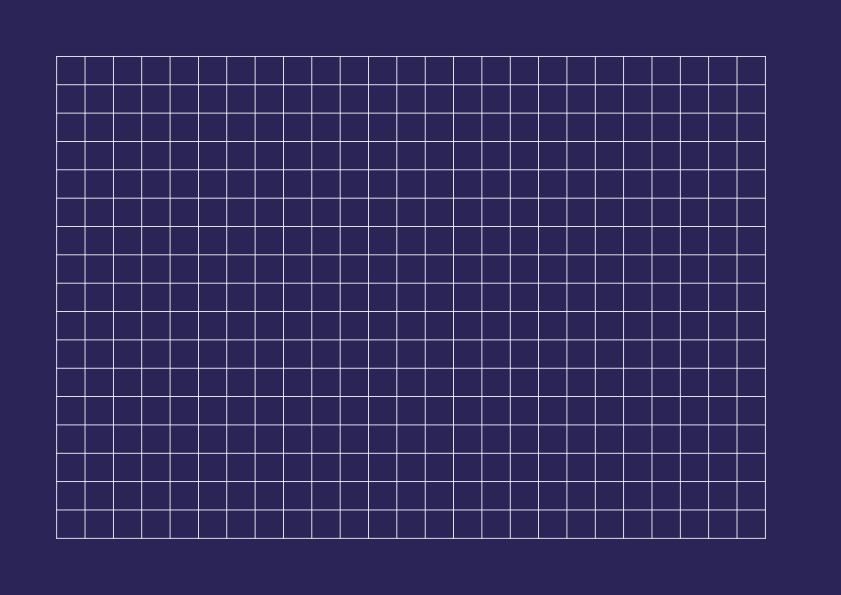# Chat GPT4 makes graph paper!

It does take an extra step, but it figured it out in python!

The Prompt:
Please write a python script using fpdf that will output a file “my-grid.pdf” which is a landscape a4 pdf file with a background color of dark purple, and has a border of at least 2cm. In the remaining space, figure out how many 1cm x 1cm squares would best fill the remaining space, and build a 1cm x 1cm grid with thin white lines. Do not draw partial squares.The Code:
`from fpdf import FPDF`

``` # Constants A4_WIDTH = 297 # Width of A4 paper in mm (landscape) A4_HEIGHT = 210 # Height of A4 paper in mm (landscape) BACKGROUND_COLOR = (41, 31, 88) # Dark purple BORDER_WIDTH = 20 # 2cm border width GRID_SIZE = 10 # 1cm x 1cm squares GRID_COLOR = (255, 255, 255) # White # Initialize PDF pdf = FPDF("L", "mm", "A4") pdf.set_auto_page_break(False) pdf.add_page() # Set background color pdf.set_fill_color(*BACKGROUND_COLOR) pdf.rect(0, 0, A4_WIDTH, A4_HEIGHT, "F") # Calculate grid dimensions grid_width = A4_WIDTH - 2 * BORDER_WIDTH grid_height = A4_HEIGHT - 2 * BORDER_WIDTH grid_columns = grid_width // GRID_SIZE grid_rows = grid_height // GRID_SIZE # Draw grid pdf.set_draw_color(*GRID_COLOR) pdf.set_line_width(0.3) # Thin lines for row in range(grid_rows + 1): y = BORDER_WIDTH + row * GRID_SIZE pdf.line(BORDER_WIDTH, y, BORDER_WIDTH + grid_columns * GRID_SIZE, y) for col in range(grid_columns + 1): x = BORDER_WIDTH + col * GRID_SIZE pdf.line(x, BORDER_WIDTH, x, BORDER_WIDTH + grid_rows * GRID_SIZE) ```

```# Save PDF pdf.output("my-grid.pdf")```

And the final PDF output (after the script is run locally)

If you run into problems at any step of the way, paste your errors into ChatGPT, and it’ll help you out!